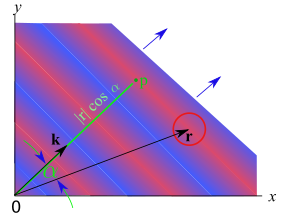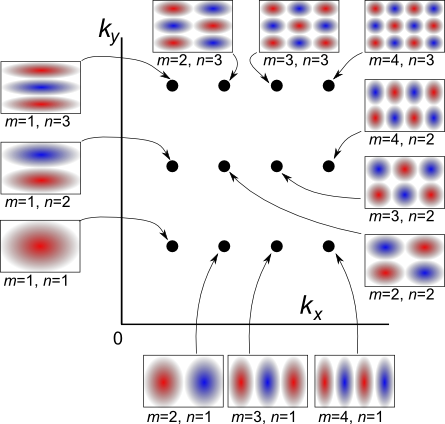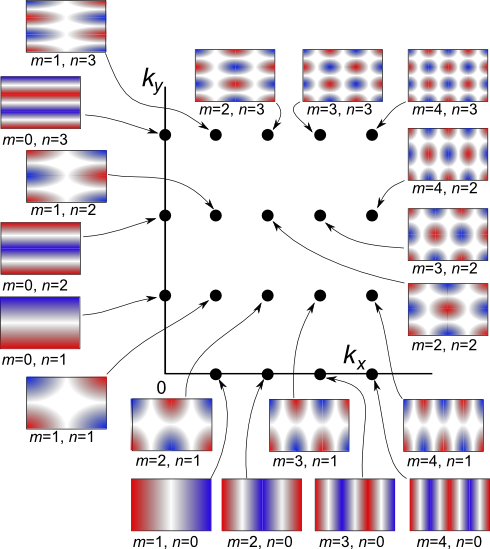There are all sorts of resonances around us, in the world, in our culture, and in our technology. A tidal resonance causes the 55 foot tides in the Bay of Fundy. Mechanical and acoustical resonances and their control are at the center of practically every musical instrument that ever existed. Even our voices and speech are based on controlling the resonances in our throat and mouth. Technology is also a heavy user of resonance. All clocks, radios, televisions, and gps navigating systems use electronic resonators at their very core. Doctors use magnetic resonance imaging or MRI to sense the resonances in atomic nuclei to map the insides of their patients. In spite of the great diversity of resonators, they all share many common properties. In this blog, we will delve into their various aspects. It is hoped that this will serve both the students and professionals who would like to understand more about resonators. I hope all will enjoy the animations.

Origins of Newton's laws of motion

History of mechanical clocks with animations
Understanding a mechanical clock with animations
includes pendulum, balance wheel, and quartz clocks

## Sunday, March 13, 2016

### Traveling and standing waves in two and three dimensions - Cartesian coordinates

 All postings by author previous: Waves in a ring resonator - standing and rotating waves up: Postings in this section next: Standing waves and rotating waves in two dimensional circular resonators

 This posting includes flash animations showing the physics discussed. Most computers have a flash player already installed, but if yours does not, download the free Adobe flash player here. Flash animations:

Keywords: standing wave modes, 2D, traveling waves, Dirichlet, Neumann boundary conditions, rotating wave, Flash animation, technical illustration

## Traveling and standing waves in two and three dimensions - Cartesian coordinates:Fig. 25. Ocean waves. Lines of gentle swells moving across of surface of the ocean. In this photo you can see both large wavelengths and distinctly shorter wavelengths moving in two different directions.

### 1. Traveling waves

Traveling waves have been covered in previous postings: , , , ,  and  and elsewhere. This posting extends the treatment to 2D and 3D Cartesian coordinate [ref] systems (x-y and x-y-z systems).

### 2. Mathematics of linear traveling waves in two and three dimensions

In a one dimensional system such as waves on a string, we express traveling waves as:

ψ(x,t) = A cos(kx − ωt)    and      ψ(x,t) = A exp i(kx − ωt)      ,     (25)

in real and complex notation styles, respectively. In an open medium there are no constraints on the frequency ω so that the frequency is determined by the wave powering device or phenomena. The wavenumber k is given by k = 2π/λ = ω/vp  where λ is the wavelength and vp is the wave's phase velocity. Use of a negative k results in waves that propagate in the negative x direction.⇑ Fig. 26a. Animation of a two dimensional traveling wave. Mouse over this figure to see the animation, mouse off to suspend it or click on it to reset it. ⇑ Fig. 26b. Illustration of traveling waves and various parameters. A portion of a traveling wave is shown in red and blue, representing the wave crests and troughs. The wavenumber vector k is shown pointing in the direction of wave propagation with a magnitude equal to 2π/λ. The blue vectors also show the direction of wave propagation. The red circle represents an arbitrary area at which a person might want to calculate the wave. The position of the red circle is given by the vector r. More on Fig. 26b: In the text just below, we replace the kx of Equation (25) with the dot product of the three dimensional vectors k and r, i.e. replace  kx  with  k⋅r . One way to view this approach is that we are effectively rotating the coordinate system so that the x axis is along the direction of wave propagation and the wave is then one dimensional because all the variation is in the new x direction (not in the new y direction), exactly the situation Equation (25) is designed to handle. The new  x  equals  |r| cosα , where x is measured in the direction of wave propagation, i.e. along the green line in Fig. 26b. For r shown, the length of the green line (from origin to point p) is the value of x. Thus because k⋅r = |k|⋅|r| cosα = kx we see that Equations (25) and (26) are equivalent.

We expand these equations to allow for linear waves in two and three dimensions by treating the wavenumber k as a vector. As shown in Fig. 26b, the vector k is pointed in the direction of propagation and has the same magnitude as in the scaler case, i.e. |k| = 2π/λ = ω/vp . We incorporate this vector wavenumber into Equation (25) as:

ψ(r,t) = A cos(k⋅r − ωt)    and      ψ(r,t) = A exp i(k⋅r − ωt)    ,   (26)

where k⋅r is the dot product of the wavenumber vector k and the position vector r:

k⋅r = kxx + kyy       and      k⋅r = kxx + kyy + kzz       ,      (27)

in two and three dimensions respectively. For example, the first equation of (26) can be written for three dimensions as:

ψ(x,y,z,t) = A cos(kxx + kyy + kzz − ωt)     .      (28)

### 3. Standing waves in two dimensions

We have just had a discussion of traveling waves in two and three dimensions. Next we will discuss standing waves as are usually found in confined rectangular spaces. As in one dimension, the mathematical form of these can be arrived at in two different ways. We can consider a sinusoidal expression of the form typical of standing waves, i.e. of the form of (29) below, or superpositions of oppositely directed traveling waves (see Box 1 for more of this approach).

One arrangement that can support standing waves that is easy to address with Cartesian coordinates is a two dimensional wave medium surrounded by a rectangular frame or walls that reflect the waves. These walls will impose boundary conditions which may require that the wave be zero at the wall (Dirichlet type) or that the spatial derivative of the wave be zero (i.e. zero gradient) at the wall (Neumann type). In this case it is useful to use solutions of the wave equation of the form:

ψ(x,y,t) = A sin kxx⋅sin kyy⋅cos ωt      and      ψ(x,y,t) = A cos kxx⋅cos kyy⋅cos ωt      ,      (29)

for the two different boundary conditions. One can show that the form of (29) can be arrived at by a sum of four waves of the form of (26) with carefully chosen vector wavenumbers (See Box 1).

Box 1. Derivation of the form of (29)
from waves of the form of (26)

We choose to add four traveling waves and with the absolute values of kx and ky satisfying (30) but with varying signs:

ψ = ei(kxx+kyy−ωt)ei(−kxx+kyy−ωt)ei(kxx−kyy−ωt)ei(−kxx−kyy−ωt)

By factoring out common terms and use of  cos φ = ½(ee)  we get:

ψ = 2 cos kxx {ei (kyy−ωt) + ei(−kyy−ωt)}

= 4 coskxx coskyy  eiωt

which is basically the same as (29b). Alternately, we could arrive at the form of (29a) by replacing two of the plus signs between major terms of our starting sum of four traveling waves with minus signs.We might also note that our choice of four traveling waves could be viewed as a traveling wave plus its three images made by the resonator walls acting as mirrors. This reinforces the idea that a wave resonator is a container for waves, that a trapped wave bounces around inside a boundary of mirrors.

Similar to the waves on a string case (one dimensional case) [ref] the upper boundary conditions require that an integer number of half wavelengths fit into both the length L and the width W of the rectangle. This means that:

kx = /L    and    ky = /W    ,    (30)

where the mode indices m and n are both integers. In the Dirichlet case, these integers must both be larger than zero. In the Neumann case one, but not both, can be zero.

The angular frequency ω is related to the wavenumbers by:

ω = k vp      where       k = √ kx2 + ky2       .      (31)

k is the magnitude of the wavenumber vector and vp is the phase velocity of traveling waves of this frequency in this wave medium.

Combining (30) and (31) we get:

ωm,n = π vp(ω) √ (m/L)2 + (n/W)2       ,      (32)

where we have emphasized that the phase velocity can be a function of the frequency (in the case of a dispersive wave medium[ref]).

Below we animate several of these modes, identified by their modal indices m and n. Mouse over these figures to see the animations, mouse off to suspend them or click on them to reset them.

Table 7. Animation of modes of a rectangular 2D resonator
⇐ Fig. 27. This animation shows the m = 1, n = 1 mode for a rectangular 2D resonator with Dirichlet boundary conditions (which require the vertical displacement to be zero at the edges). This mode is the lowest frequency mode for a Dirichlet boundary. One might call this the trampoline mode.
⇐ Fig. 28. A higher order Dirichlet mode with m = 3, n = 2.   (3 half wavelengths in the x direction and 2 half wavelengths in the y direction.)
⇐ Fig. 29. This animation shows the m = 1, n = 0 mode for a rectangular 2D resonator with Neumann boundary conditions (which require the gradient of the vertical displacement to be zero at the edges). This mode is the lowest frequency mode for a Neumann boundary. This mode could be seen as water slushing back and forth in a rectangular swimming pool or channel.
⇐ Fig. 30. A higher order Neumann mode with m = 4, n = 2.   (4 half wavelengths in the x direction and 2 half wavelengths in the y direction.)
Table 8. Wavenumber chart of modes of a rectangular resonator with Dirichlet boundary conditions.There are many more modes for a rectangular resonator than we have shown above (actually an infinite number). We show the lower frequency modes for a Dirichlet boundary condition on a wavenumber chart (Table 8 at the right). In this chart, each mode is plotted as a dot with its wavenumber ky versus its wavenumber kx. We have also added little illustrations of the modes plotted, each labeled with its indices m and n. While this chart is static, each mode is understood to oscillate vertically as do the animations in Table 7 above.

At the bottom of the wavenumber table we see a linear graph showing the frequencies of the modes using Equation (32), assuming that L = 1, W = 0.68, and vp = 2. The numbers beside each "line" are the mode indices, i.e. m,n for the particular mode. Equation (32) also indicates that the frequency of each mode is proportional to the magnitude of the wavenumber vector k = √ kx2 + ky2 , meaning that the frequencies are proportional to the distance a mode is from the origin on the wavenumber chart.

Looking at Equation (30) above, we see that the modes on the wavenumber chart have a horizontal spacing inversely proportional to the resonator's length L (measured in the x direction) and a vertical spacing inversely proportional to the resonator's width W (measured in the y direction). Since for the resonator shown W = 0.68L, i.e. L is larger than W, the modes are more tightly spaced in the x direction than the y direction.

Table 9 is similar to Table 8 except that it is for a rectangular resonator with Neumann boundary conditions. The illustrations are a little different and also there are additional modes ... those with indices containing a zero. Other than these zero index modes, the placement of the modes in wavenumber space is the same in the two tables. Also note that the modes having a zero as one of their indices are basically one dimensional, that all the variation takes place along the direction associated with the non-zero index.

Table 9. Wavenumber chart of modes of a rectangular resonator with Neumann boundary conditions.We might also point out that irregular 2D shapes (such as the heart shaped resonator) also have similar modes to rectangular resonators although these are not so easy to calculate as for rectangles. In the next posting, we will look at 2D resonances for circular resonators.

 Fig. 31. Rotating mode in a square resonator with Neumann boundary conditions.

### 4. Rotating mode in a square resonator

In the case of a square resonator with Neumann boundary conditions, we can excite a rotating mode by using a sum of two standing wave modes: the mode  m = 0, n = 1  and also the mode m = 1, n = 0 with one of the modes delayed in time by π/2 radians. We see an animation of this in Fig. 31. Mouse over the figure to see the animations, mouse off to suspend it or click on it to reset it.

### 5. Standing waves in three dimensions

We might also point out that similar to the 2D rectangular resonators discussed above, resonances can also occur inside three dimensional boxes and the math is almost the same as in the two dimensional case:

ψ(x,y,z,t) = A sin kxx⋅sin kyy⋅sin kzz⋅cos ωt      and      ψ(x,y,z,t) = A cos kxx⋅cos kyy⋅cos kzz⋅cos ωt      ,      (33)

for the Dirichlet and Neumann cases respectively. Equations (30) through (32) also need to have a z component added for the 3D box case.

 All postings by author previous: Waves in a ring resonator - standing and rotating waves up: Postings in this section next: Standing waves and rotating waves in two dimensional circular resonators